### Ian D. Jonsen, Joanna Mills Flemming, and Ransom A. Myers. 2005. Robust state–space modeling of animal movement data. Ecology 86:2874–2880.

Appendix B. Data regularization procedure and comparison of movement parameters derived from the regularized hooded seal data and those derived from state estimates from the DCRW model fit to the hooded seal data.

A useful feature of the state estimates obtained from our analyses is that they can be used to derive fundamental descriptors of movement behavior such as turning angles and movement rates. In order to evaluate our approach in this regard we compare turning angles and rates of movement derived from state estimates (obtained via the DCRW model) to those obtained using the data directly. Note that for the latter approach, one must first regularize the data to obtain locations at regular time intervals since behavioral parameters are only meaningful when derived either from a series of known start and end locations (we can not observe these with remote sensed data) or from a series of locations at regular time intervals (Turchin 1998).

Here we describe the data regularization procedure. Suppose we have a series of observed locations,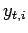, sampled irregularly through time, where t represents the regular time interval and i indexes the observations within each interval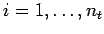. By calculating a weighted average of the locations observed within an interval, we obtain an estimate of location for each time interval. This collection of estimates is then regularly spaced in time.

To obtain the regularized data presented in Fig. B1, we maximize the following likelihood for regular time intervals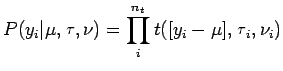where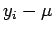is the average location,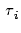and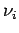are the scale and df estimates, respectively, associated with the quality class for each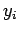. We fixandat the values presented in Appendix A (Table A1), leavingto be estimated.

Prior to conducting this regularization of the data, we removed the three extreme locations indicated in Fig. 1A. We fit wrapped Cauchy and Weibull distributions (e.g., Morales et al. 2004) to the turning angles and movement rates, respectively.

The distributions of turning angles calculated from the regularized data and from the state estimates differ little from one another, although the state estimates suggest a slightly broader distribution with more course reversals and a mean turning angle that favours turns to the left (Fig. B1). The movement rate distributions differ more substantially; the state estimates yield a larger mode and narrower distribution overall (Fig. B1). We have more confidence in the movement parameters derived from the state estimates because the uncertainty in the observations has been dealt with in a more complete manner, ie. both estimation error and process variability are accounted for, whereas the regularization procedure accounts only for estimation error.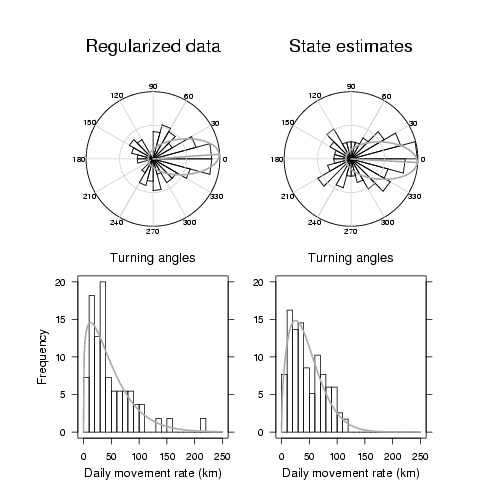FIG. B1. Comparision of turning angle and movement rate distributions derived from regularized data and from state estimates. Turning angle distributions are displayed as circular histograms with wrapped Cauchy density estimates displayed as gray elipsiods. Mean turning angles are displayed as gray lines. Weibull density estimates are overlaid on the movement rate histograms (gray curves).

LITERATURE CITED

Morales, J. M., D. T. Haydon, J. Friar, K. E. Holsinger, and J. M. Fryxell. 2004. Extracting more out of relocation data: building models as mixtures of random walks. Ecology 85:2436–2455.

Turchin, P. 1998. Quantitative Analysis of Movement: Measuring and modeling population redistribution in animals and plants. Sinauer Associates, Sunderland, Massachusetts, USA.

[Back to E086-156]